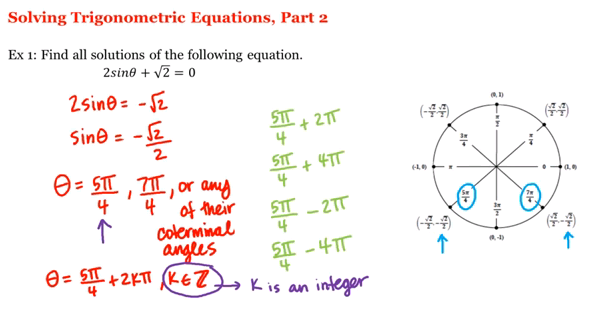Home

Math 1A/1B. Pre-Calculus: Solving Trigonometric Equations, Part 2 (English)

UCI Math 1A/1B: Pre-Calculus
Pre-Calculus: Solving Trigonometric Equations, Part 2
View the complete course: http://ocw.uci.edu/courses/math_1a1b_precalculus.html
Instructor: Sarah Eichhorn, Ph.D and Rachel Lehman, Ph.D

More courses at http://ocw.uci.edu

Description: UCI Math 1A/1B: Precalculus is designed to prepare students for a calculus course. This course is taught so that students will acquire a solid foundation in algebra and trigonometry. The course concentrates on the various functions that are important to the study of the calculus.

Required attribution: Eichhorn, Sarah; Lehman, Rachel Pre-calculus 1A/1B (UCI OpenCourseWare: University of California, Irvine), http://ocw.uci.edu/courses/math_1a1b_precalculus.html. [Access date]. License: Creative Commons Attribution-ShareAlike 4.0 United States License.Author:
Sarah Eichhorn
Title:
Lecturer
Department:
Mathematics
Author:
Rachel Lehman
Title:
Lecturer
Department:
Mathematics